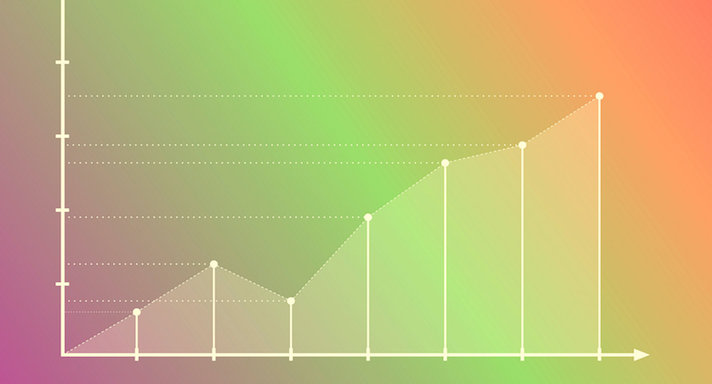# Basic Math Concepts II for High School### Course Overview

Basic Math Concepts II for High School is recommended for high school students with weak math skills. It presents the lesson material on a 5th grade level. This course covers basic math operations with whole numbers, fractions, and decimals, and introduces algebraic expressions. Basic Math Concepts II for High School is taught by Acellus Instructor Trisha Madden.This course was developed by the International Academy of Science. Learn More

### Scope and Sequence

Unit 1 In this unit students study addition and place value. They review subtraction and decimals, and explore multiplication and division. Unit 2 In this unit, students focus on decimals, including rounding, sums and differences, and estimation, and they study the same things as they relate to whole numbers. They explore the associative and commutative properties and multiple-step story problems. Unit 3 In this unit students delve into a study of multiplication, using one- and two-digit numbers, and larger numbers. They also study the properties of multiplication, estimating products, and mental math. Unit 4 In this unit students focus on estimation. They discuss reasonableness, and explore dividing tens and hundreds, as well as zero-containing quotients. Unit 5 In this unit students continue to explore estimation skills. They also study strategies for division, math in real-world applications, and quotients of differing sizes. Unit 6 Through a discussion of expressions, variables, order of operations, and expressions with brackets, students begin in this unit to learn about algebra. They also study numerical patterns. Unit 7 In this unit students explore the multiplication of decimals by whole numbers, by other decimals, and by powers of ten. They also review estimating products. After this unit students are presented with the Mid-term Review and Final. Unit 8 In this unit students delve into the division of decimals by whole numbers and by powers of ten. They explore estimating quotients and exponents, multiple-step problems from the real work, and decimals as divisors and as dividends. Unit 9 Through the study of squares, rectangles, quadrilaterals, angles, and triangles, students are introduced in this unit to the subject of geometry. Included in this study are measuring and classifying. Unit 10 In this unit students build foundational knowledge of fractions, exploring simplest form, equivalent fractions, and mixed numbers. They also study division with fractions, and changing fractions to decimals. Unit 11 In this unit students expand their understanding of fractions with addition and subtraction, least common multiples, like denominators, and estimation using benchmark fractions. They also study least common denominators. Unit 12 In this unit students focus on adding and subtracting mixed numbers. They also explore estimating mixed numbers. Unit 13 In this unit students further explore fractions, focusing on multiplying and dividing fractions, as well as whole numbers and mixed numbers. They also study using fractions to find the area of a rectangle, as well as estimation. Unit 14 In this unit students explore the volume of solid figures. They also study modeling volume of objects, both regular and irregular. Unit 15 In this unit students study customary and metric units. They explore mass, weight, capacity, and length. They practice converting measurement units, and do story problems, including multiple-step story problems. Unit 16 In this unit students investigate graphs and line plots. They also study ordered pairs, comparing numerical patterns, solving problems, data collection, interpreting patterns, and surveys. Following this unit, students are presented with the Final Review and Exam.